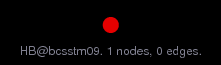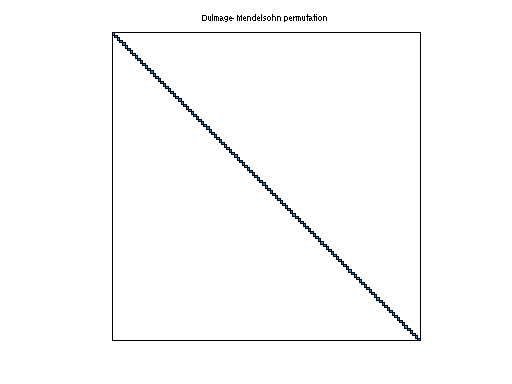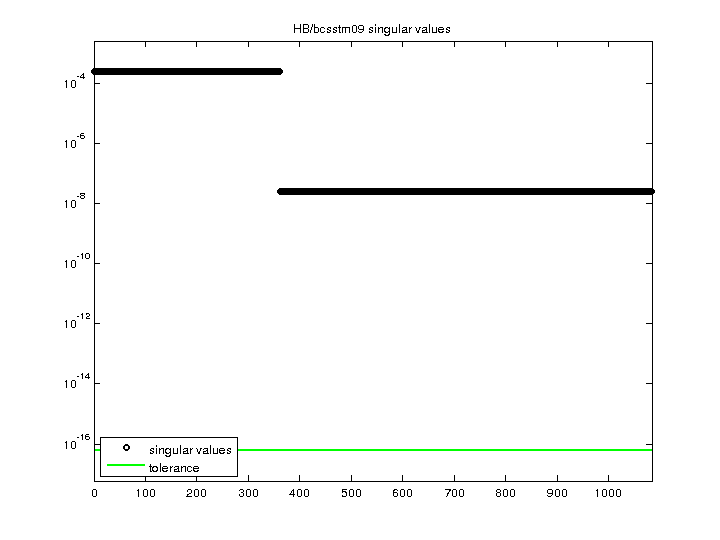Matrix: HB/bcsstm09

Description: SYMMETRIC MASS MATRIX, SQUARE PLATE CLAMPED(undirected graph drawing)• Matrix group: HB
• download as a MATLAB mat-file, file size: 2 KB. Use UFget(64) or UFget('HB/bcsstm09') in MATLAB.

 Matrix properties number of rows 1,083 number of columns 1,083 nonzeros 1,083 structural full rank? yes structural rank 1,083 # of blocks from dmperm 1,083 # strongly connected comp. 1,083 explicit zero entries 0 nonzero pattern symmetry symmetric numeric value symmetry symmetric type real structure symmetric Cholesky candidate? yes positive definite? yes

 author J. Lewis editor I. Duff, R. Grimes, J. Lewis date 1982 kind structural problem 2D/3D problem? yes

 Ordering statistics: result nnz(chol(P*(A+A'+s*I)*P')) with AMD 1,083 Cholesky flop count 1.1e+03 nnz(L+U), no partial pivoting, with AMD 1,083 nnz(V) for QR, upper bound nnz(L) for LU, with COLAMD 1,083 nnz(R) for QR, upper bound nnz(U) for LU, with COLAMD 1,083

 SVD-based statistics: norm(A) 0.00025902 min(svd(A)) 2.5902e-08 cond(A) 10000 rank(A) 1,083 sprank(A)-rank(A) 0 null space dimension 0 full numerical rank? yes

 singular values (MAT file): click here SVD method used: s = svd (full (A)) ; status: ok